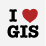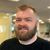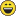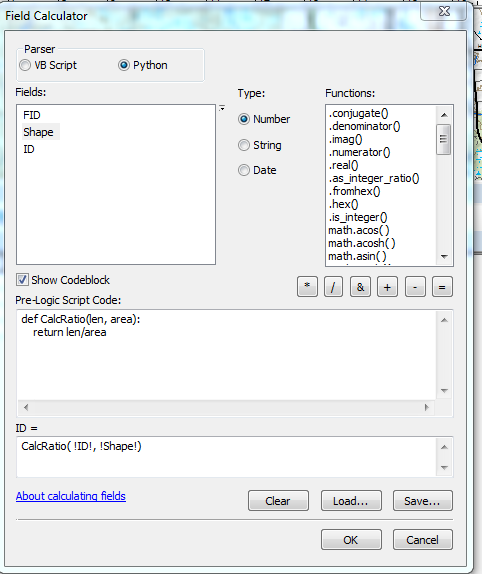# Calculate polygon area perimeter ratio

3802
4
10-14-2014 01:57 PMNew Contributor

I want to write a python script to calculate the edge to area ratio of each county in mi_counties.shp (Shape_Leng / Shape_Area). What would be the script look like with expression to calculate the ratio? Please help. Thank you!

(Curtis Price edited title, was "Hi")

Tags (5)
1 Solution

Accepted SolutionsbyEsri Regular Contributor

This can be done using the geometry column of the shapefile to return the length and area in the Calculate Field tool.

```import arcpy

shp = r"<Path>\<ShapefileName>.shp"

arcpy.CalculateField_management(in_table=shp,
field="EdgeRatio",
expression="!shape.length!/!shape.area!",
expression_type="PYTHON_9.3",
code_block="#")
```
4 RepliesbyEsri Regular Contributor

This can be done using the geometry column of the shapefile to return the length and area in the Calculate Field tool.

```import arcpy

shp = r"<Path>\<ShapefileName>.shp"

arcpy.CalculateField_management(in_table=shp,
field="EdgeRatio",
expression="!shape.length!/!shape.area!",
expression_type="PYTHON_9.3",
code_block="#")
```by
Regular Contributor III

Ok, well sure, beat me to a more elegant solution. The only caveat is if the OP isn't calculating a field, but getting the results for another process, as part of a more complex script. But your answer seems the most likely and best.by
Regular Contributor III

Hi Narayan. The script you want, or at least the formula you gave, is pretty simple.

```def CalcRatio(len, area):
return len/area
```

The differences in how you call this depend on whether you want to call this in Field Calculator or a stand alone script.

In FC, set the parser to python and check the show codeblock box. Enter the above code in the pre-logic box, and in the field = box  call the function as shown below (obviously use your own field names, not my example).You'd use the same def in a stand alone script, but the method for getting the field values for length and area are a little different. If that's what you want, post back and we can go through that.New Contributor

Greg and Christian, thank you so much! I will try using both of these codes and let you know later. Thank you again.Greg Keith‌ and Christian Wells